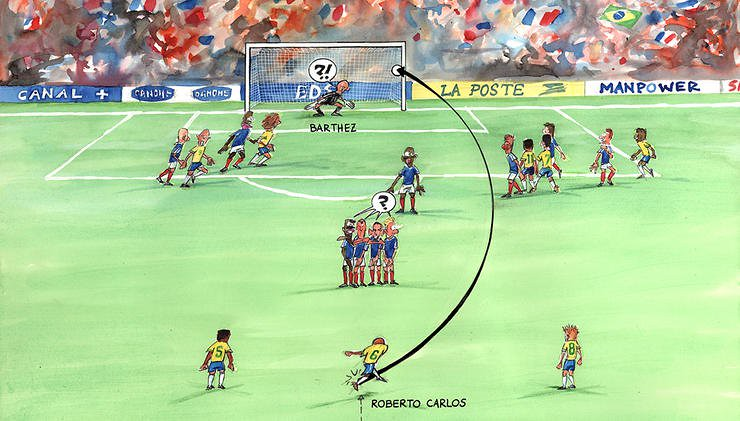Select Page
##### By Sophia Eiley

Many of the extraordinary goals in football history — from the likes of our idols such as Messi, Ronaldo and DeBruyne, as well as Roboerto Carlos’ banana swerve screamer in the France 1997 world cup — are owed to physics.Source: https://images.app.goo.gl/uRd17ESNw1kudLWaA

The Magnus force is the pressure differential that gradually curls the ball; the generation of force perpendicular to the ball spinning in the air. The phenomenon is a result of Newton’s third law which states that every action will have an equal and opposite reaction. When kicked, the force the foot exerts on the ball is equal to the force the ball imposes on the air. Because the ball pushes the air in one direction, the air propels the ball in the opposite direction. Airstreams are pulled under the ball by its rotation while the force stays perpendicular to the velocity vector of the ball. There is an imbalance of pressure, as the tangential velocity of the ball on the top side is opposite the airflow, and the tangential velocity of the bottom half follows the direction of the airflow. As the ball loses velocity, the Magnus effect becomes more prominent. As the airflow moving against the ball stops, the pressure imbalance increases. A force acts downward and to the side of the ball that has the opposing airflow, causing it to curve. If the ball is kicked with a counter-clockwise spin, the Magnus force acts on the right of the ball causing it to curve right. The equation for the Magnus force is Fm = S (w * v). Where Fm is the Magnus force vector, S is the air resistance coefficient across the surface of the ball, w is the angular velocity vector of the ball and v is the velocity of the fluid (air). When the Magnus force is found, basic kinematic equations can be used to predict the characteristics of spinning objects in flight.a) Diagram demonstrating the relationship between airflow, spin and curve by showing that a ball spinning in a counter-clockwise direction will curve right due to the imbalance of pressure and tangential velocity, which occurs because the right side of the ball travels against the airflow and the left follows it.

Newton’s first law states that an object at rest will stay at rest unless acted upon by an external force and an object in motion will stay in motion unless acted upon by an external force. The player’s foot moves the ball, and the forces slowing the ball down while it moves through the air include drag and friction caused by wind and gravity. As the ball moves in the air, it experiences resistance called aerodynamic drag, making it appear still with the air moving past the object at speed. Air molecules create a thin layer around the surface of the ball and pull in the surrounding airflow. The relative strength of the inertial (momentum) and viscous forces in the flow determine airflow and the value of drag of the ball. To calculate the ratio between the inertial and viscous force, we use the Reynolds number: Re = rho * V * d / mu, where rho is air density, V is velocity, d is diameter of the ball, and mu is air viscosity. If the Reynolds number is low, then the viscous force dominates the inertial force creating a thick laminar boundary layer on the surface of the ball. If the Reynolds number is higher, then the boundary layer is thin because the interior forces dominate the viscous force. Another influence on an object’s drag is the size, shape, and atmospheric conditions. The following equation is used to predict drag: D = .5 * rho * V^2 * A * cd, where d is drag, A is the cross-sectional area, and cd is the drag coefficient. Drag is produced by the boundary layer separating from the back of the ball. As it separates, a viscous wake forms behind the ball. A large wake creates a large amount of drag and a thin wake creates less drag. The drag on the ball (the thickness of the wake) is determined from the conditions of the boundary layer which comes from the Reynolds number. At low Reynolds values, the boundary layer is laminar and the wake is thick. At higher Reynolds numbers, the boundary layer is turbulent, which initially produces a thin wake. When the ball increases in speed and therefore the Reynolds number increases, the wake thickens and drag increases.b) Diagram illustrating how a ball travelling at a low speed has a larger airflow separation and boundary layer, therefore creating a large viscous wake.c) Diagram illustrating how a ball travelling at a high speed has a smaller airflow separation and boundary layer, therefore creating a small viscous wake.

While I highly doubt that football players go out of their way to learn all the phenomena that make their goals possible, from practice, repetition, and ball sense, these athletes are quite the physics experts. They know how to place the ball in parts of the net while utilizing these phenomena to make their spectacular kicks possible.

Sophia is a grade 10 student at St. Michaels University School in British Columbia. She spends her time playing soccer and tinkering as a part of her school’s robotics team. In her future, Sophia plans to pursue mechanical engineering in post secondary education.

##### Works Cited:

 Hall N, editor. Drag on a soccer ball [Internet]. NASA. NASA; 2021 [cited 2022Mar29]. Available from: https://www.grc.nasa.gov/www/k-12/airplane/socdrag.html

 Brandon P. What is the Magnus effect and how to calculate it: [Internet]. What is the Magnus effect. [cited 2022Mar29]. Available from: http://ffden-2.phys.uaf.edu/211_fall2010.web.dir/Patrick_Brandon/what_is_the_magnus_effect.html

 Peshin A. How footballs, ping-pong, tennis and basketballs swerve in mid-air? [Internet]. Science ABC. 2022 [cited 2022Mar29]. Available from: https://www.scienceabc.com/pure-sciences/what-is-the-magnus-effect-swerve-ball-basketball.html